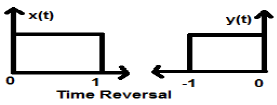# DSP - Operations on Signals Reversal

#### Digital Marketing Online Training

23 Lectures 2 hours

#### How To Start Your Own Digital Marketing Agency

125 Lectures 9 hours

#### Digital Marketing Agency Elite Consultants Masterclass

140 Lectures 20 hours

Whenever the time in a signal gets multiplied by -1, the signal gets reversed. It produces its mirror image about Y or X-axis. This is known as Reversal of the signal.

Reversal can be classified into two types based on the condition whether the time or the amplitude of the signal is multiplied by -1.

## Time Reversal

Whenever signal’s time is multiplied by -1, it is known as time reversal of the signal. In this case, the signal produces its mirror image about Y-axis. Mathematically, this can be written as;

$$x(t) \rightarrow y(t) \rightarrow x(-t)$$

This can be best understood by the following example.In the above example, we can clearly see that the signal has been reversed about its Y-axis. So, it is one kind of time scaling also, but here the scaling quantity is (-1) always.

## Amplitude Reversal

Whenever the amplitude of a signal is multiplied by -1, then it is known as amplitude reversal. In this case, the signal produces its mirror image about X-axis. Mathematically, this can be written as;

$$x(t)\rightarrow y(t)\rightarrow -x(t)$$

Consider the following example. Amplitude reversal can be seen clearly.